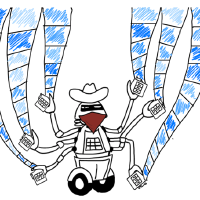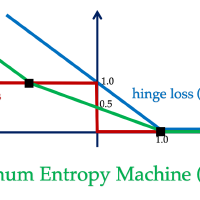# Machine Learning & Statistics

We take real-world problems, abstract mathematical models from them, and develop algorithms using first principle approaches. We consider a wide range of topics in machine learning and statistics, including classification, clustering, multi-armed bandits, deep learning, empirical Bayes, multiple hypothesis testing.### Adaptive Monte Carlo Computation

In modern data science, datasets are growing at a tremendous speed, making many commonly-used algorithms computationally expensive. To accelerate existing algorithms, we consider a general idea called adaptive Monte Carlo computation. At a high level, it contains two steps: 1) convert the computational problem into a statistical estimation problem; 2) accelerate the estimation by designing an adaptive sampling procedure via multi-armed bandits. In a few applications, applying such an idea brings an improvement of several orders of magnitude in wall clock time.

Such a procedure has witnessed a few successes. Med-dit accelerates medoid identification, where the medoid of a set of n points is the point in the set with the smallest average distance to other points. AMO considers optimizing an arbitrary objective function over a finite set of inputs, covering several important applications including nearest neighbor, hierarchical clustering, and feature selection. Also, AMT accelerates the standard Monte Carlo multiple testing workflow. In all these applications, applying the idea of adaptive Monte Carlo computation brings an improvement of several orders of magnitude in wall clock time.

#### Key Publications

• “Adaptive Monte Carlo Multiple Testing via Multi-Armed Bandits”, Martin J. Zhang, James Zou, David Tse, 2019, arXiv 1902.00197, ICML 2019
• “Adaptive Monte-Carlo Optimization”, Vivek Bagaria, Govinda M. Kamath, David N. Tse, 2018, arXiv 1805.08321
• “Medoids in almost linear time via multi-armed bandits”, Vivek Bagaria, Govinda Kamath, Vasilis Ntranos, Martin Zhang, David Tse, 2018, AISTATS 2018### Deep Learning

Deep learning algorithms have achieved state-of-the-art performance over a wide range of machine learning tasks. However, the current theoretical understanding of their success cannot explain the robustness and generalization behavior of deep learning models. We seek to analyze and improve approximation, generalization, and stability properties of deep learning algorithms.

Despite their practical success in benchmark computer vision and natural language processing tasks, the theoretical aspects of deep learning algorithms are poorly understood. For example, why do neural net classifiers generalize well for the true labels of image data while they can easily overfit randomly-generated labels? How does a stochastic gradient optimization algorithm manage to converge to generalizable local minima? Why are state-of-the-art neural net classifiers vulnerable to minor adversarial perturbations and how can we improve the robustness properties of deep learning algorithms? We aim to understand the answers to these questions and design mechanisms for improving generalization and robustness properties of deep learning models. We use tools and frameworks from information theory, optimal transport theory, and convex analysis to address the above questions.

#### Key Publications

• “Generalizable Adversarial Training via Spectral Normalization”, Farzan Farnia, Jesse M. Zhang, David Tse, 2018, arXiv 1811.07457, ICLR 2019, Code, Poster
• “Porcupine Neural Networks: (Almost) All Local Optima are Global”, Soheil Feizi, Hamid Javadi, Jesse Zhang, David Tse, 2017, arXiv 1710.02196, NeurIPS 2018, Code, Poster### Maximum Entropy Framework for Supervised Learning

Minimizing empirical 0-1 loss for classification tasks is known to be an NP-hard problem. In this project, we formulate a minimax learning problem which optimizes the worst-case 0-1 loss over an ambiguity set around the empirical distribution of data. We provide interpretation and efficiently solve the formulated minimax problem by connecting its dual problem to maximizing the entropy function.

Supervised learning, the task of predicting the label of an unseen data-point using the knowledge of some training samples, is a central problem in machine learning. Minimizing the empirical risk over a hypothesis set, called empirical risk minimization (ERM), is commonly considered as the standard approach to supervised learning. However, ERM applied to non-convex loss functions such as 0-1 loss leads to intractable optimization problems. To resolve this issue, 0-1 loss is usually replaced by a convex surrogate loss such as cross-entropy loss in the logistic regression and hinge loss in the support vector machines (SVMs). In this project, we develop a minimax approach to supervised learning by finding the minimax optimal prediction rule optimizing the worst-case 0-1 loss over an ambiguity set around the empirical distribution of training data. We leverage convex analysis and information theory tools to reduce the minimax problem to a convex optimization task. This approach results in a novel classification algorithm which we call the maximum entropy machine (MEM). We connect the MEM classification problem to the maximum entropy principle, showing it reduces to maximizing a generalized conditional entropy function over the ambiguity set of distributions.

#### Key Publications

• “A Minimax Approach to Supervised Learning”, Farzan Farnia, David Tse, 2016, arXiv 1606.02206, NIPS 2016
• “Discrete Renyi Classifiers”, Meisam Razaviyayn, Farzan Farnia, David Tse, 2015, arXiv 1511.01764, NIPS 2015### Generative Adversarial Nets (GANs)

Generative adversarial network (GAN) is a minimax game between the generator and discriminator players. While generative models learned by GANs achieve great success in practice, GANs’ approximation, generalization, and optimization properties are still poorly understood. We focus on analyzing these theoretical aspects of GANs, providing both a comprehensive analysis addressing all the three aspects for a simplistic GAN architecture and a more specific analysis addressing only the approximation aspect for general GAN problems.

Generative adversarial network (GAN) is a minimax game between two players: a generator whose goal is to produce real-like samples and a discriminator whose task is to distinguish between the fake samples generated by the generator and the real training samples. While GANs result in state-of-the-art generative models in several benchmark computer vision tasks, their theoretical aspects are still poorly understood. Theoretical studies of GANs aim to address three main questions: 1) Approximation: Which probability distributions can be expressed by a generator function? 2) Generalization: Do the generative models learned by GANs generalize properly to the true distribution of data? 3) Optimization: Are standard gradient methods used to train GANs globally stable? We provide a comprehensive analysis of all the three aspects for a simplistic GAN formulation with linear generator and quadratic discriminator architectures. We also develop a convex analysis framework for specifically analyzing GANs’ approximation properties, which is applicable to a broad class of GAN formulations.

#### Key Publications### Covariate-adaptive multiple hypothesis testing

In multiple hypothesis testing, the data for each test boils down to a p-value while additional information is often available for each test, e.g., the functional annotation for genetic testing. Such information may inform how likely the small p-values are to be due to noise. Covariate-adaptive multiple testing methods utilize such information to improve detection power while controlling false positives.

Multiple hypothesis testing is an essential component of modern data science. Its goal is to maximize the number of discoveries while controlling the fraction of false discoveries. In many settings, in addition to the p-value, additional information/covariates for each hypothesis are available. For example, in eQTL studies, each hypothesis tests the correlation between a variant and the expression of a gene. We also have additional covariates such as the location, conservation, and chromatin status of the variant, which could inform how likely the association is to be due to noise. However, popular multiple hypothesis testing approaches, such as Benjamini-Hochberg procedure (BH) and independent hypothesis weighting (IHW), either ignore these covariates or assume the covariate to be univariate. We develop covariate-adaptive multiple testing methods, NeuralFDR and AdaFDR, to adaptively learn the optimal p-value threshold from covariates to significantly improve detection power while controlling the false discovery proportion.

#### Key Publications

• “AdaFDR: a Fast, Powerful and Covariate-Adaptive Approach to Multiple Hypothesis Testing”, Martin J. Zhang, Fei Xia, James Zou, 2018, bioRxiv 496372v1, Best paper award in RECOMB 2019
• “NeuralFDR: Learning Discovery Thresholds from Hypothesis Features”, Fei Xia, Martin J. Zhang, James Y. Zou, David Tse, 2017, NIPS 2017